﻿ Quantum computer (qubit, Josephson, ion)

# Quantum computer (= superposition ) is an illusion.

## Present quantum computer has NO future.

### [ What is a quantum computer ? ]

Quantum computer ?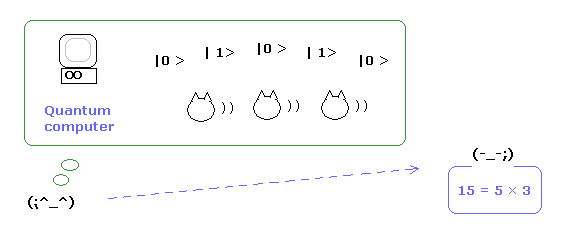You may oftern hear that quantum computers based on quantum superposition have excellent potential to compute much faster than usual classical computers.

But in fact, the present quantum computer can only do very simple factorization 15 = 5 × 3.
And this factorization has NO relations with entanglement and "superposition", as I explain later.
( This mechanism can be explained by the usual classical mechanics such as magnetic moment interactions. )

(Fig.1) Classical computer ?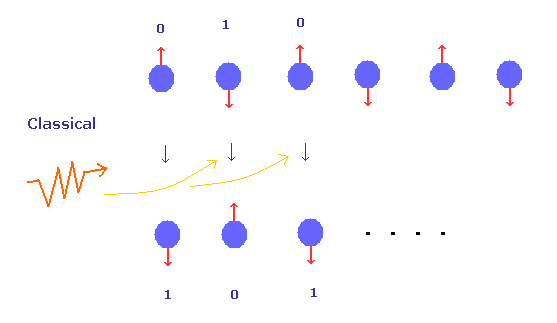In Fig.1, N particles are lining up. Each number "0" or "1" represents the particle's state such as two different energy levels, and directions of magnetic moment. ( This two different states is called "qubit" or "quantum bit". )
In classical mechanics, we have to manipulate each particle to change their numbers.
So, N manipulations (= here irradiation with some laser ) are needed.

## Superposition = Many-worlds is the heart of quantum computer.

(Fig.2) Superposition = parallel computing ??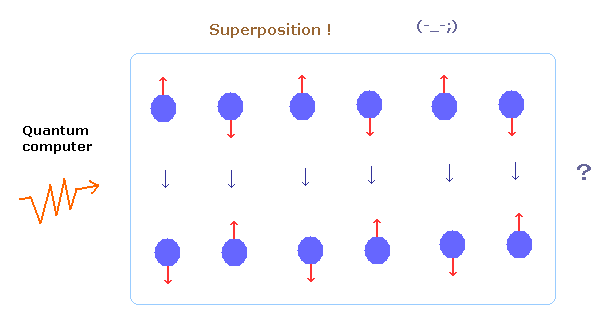According to the quantum mechanics, all particles are in mysterious superposition state.
So if we do only one manipulation, all particles can be changed at the same time ! (= parallel computing. )

They often insist this logic, but they have NO quantum computers which have achieved this supernatural computing.
And ONLY a word of "quantum computer" and algorithms have developed a life of their own, unaccompanied by experiments.

## Quantum computer can be explained by classical mechanics.

(Fig.3) Superposition = just oscillating classically.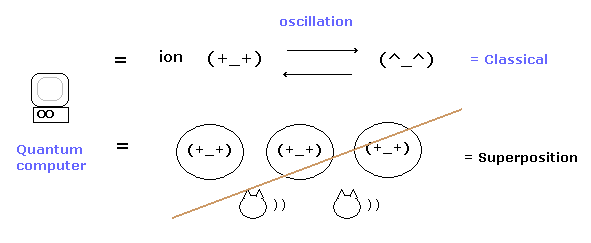There are various quantum devices (= qubits ) such as ions, Josephson juctions, NMR and Mach-Zehnder interferometer.
But all of them have reached their limits now, and have NO power of computing at all, as I explain later.
( Only NMR using fluorine can do factorization 15 = 5 × 3, but this method is based on usual classical mechanics such as magnetic moment interactions. )

The important points is that all these quantum devices cannot demonstrate the power of mysterious superposition.

For example, they only periodically change the energy states of the qubits by electromagnetic irradiation.
And they only insist classically mixed states are mysterious superpositions, because these states can be produced by usual classical manipulations.

## Two "shut up and calculate !" obstruct the computer's breakthrough.

### [ Only strange many world interpretation can explain "superposition" naturally. ]

(Fig.4) Superposition = unrealistic Many-worlds !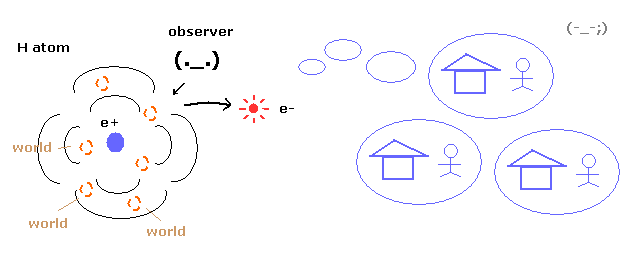If we try to realize quantum computers based on mysterious "superposition", we MUST know first what the superposition of wavefunctions really is.
Because if this superposition itself is wrong, it means we are aiming at just some illusions.

According to the quantum mechanics, the instant we try to observe a particle, the wavefunction spreading all space collapses into a point particle.

This process is faster-than-light (= nonlocal ), so we have to adopt strange many-world theory to avoid nonlocal phenomena.
In the many-world theory, observing the particle means we see one of infinite worlds which include particles of different states.

## Interference of a single particle = "real" de Broglie wave.

(Fig.5) A single C60 buckyball interferes with itself !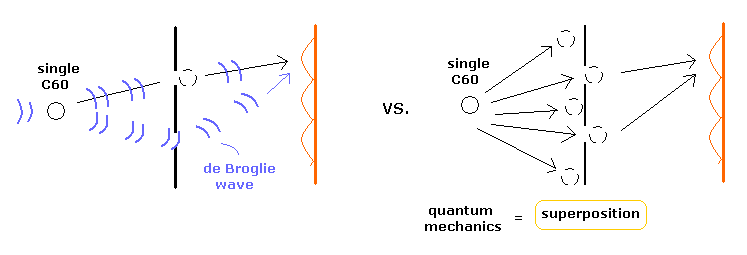Even a single C60 buckyball molecule can interfere with itself.
To explain this interference of a single particle in the double-slit experiment, the quantum mechanics needs "many-world" interpretation (= "superposition" ) to avoid faster-than-light collapse.

Of course, many world theory needs every possible state, in which worlds are unrealistically increasing like ∞ × ∞ × ∞...
So this theory is impossible, and too good to be true about the wavefunction collapse mechanism.

Bohr model, which is based on real de Broglie wave, can explain this interference naturally, as shown in Fig.5 left.
There is also "pilot-wave" theory (= Bohm interpretation ), which insists "real" particle's motion even in the quantum mechanics.

## Pilot-wave (= Bohm ) theory contradicts the history of quantum mechanics.

(Fig.6) Pilot-wave theory cannot explain electron's motion of various atoms.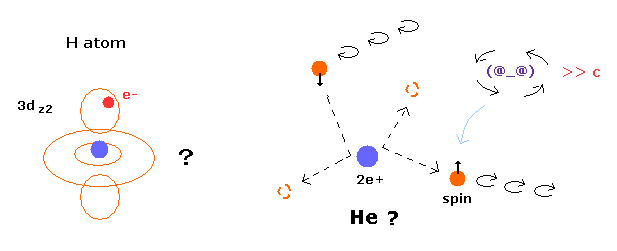Fig.6 left shows the probability density of 3d state in the hydrogen atom.
Of course, Schrodinger's hydrogen depends on Coulomb relation, so the electron MUST obey Coulomb relation.
But if the electron of 3d state is moving obeying Coulomb force, it cannot move like complicated Fig.6 left.

Furthermore, pilot wave theory cannot explain two-electron helium at all.
Because two repulsive electrons of helium are easily chaotic and autoionized.

As shown on this page, they could NOT express three-body helium using real electron's motion, so all they could choose was to accept problematic probability concept.
( If there was only hydrogen atom, Bohr-Sommerfeld model could do more naturally. )

## Pilot-wave theory = superluminal signal.

(Fig.7) Pilot-wave theory = faster-than-light signal.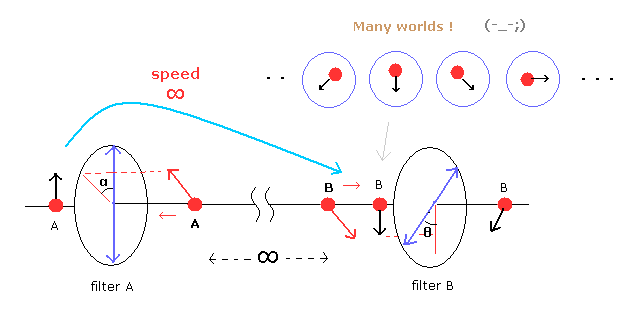You may often hear that Bell inequality violation denied hidden variable proposed by Einstein.
In Fig.7, the moment a photon (= A ) passes through the filter A and turns its polarization in the direction of filter A, the polarization of photon B also becomes the same as filter A, no matter how far they are apart from each other.
So this signal between two entangled photons travels faster-than-light (= nonlocal ).

To avoid this faster-than-light signal, they try to rely on many-world interpretation.
If we adopt "real" pilot-wave theory, we MUST accept faster-than-light signal as reality.

As shown on this page, if we use the classical electromagnetic waves, we can explain this violation as local and realistic phenomenon.
( Of course, giving up particle photon means quantum mechanics and QED themselves are wrong. )

## The most troublesome is "Shut up and calculate !" interpretaion.

(Fig.8) All interpretations are unnatural → "Shut up and calculate !"People who insist some concrete interpretations such as many-world or pilot wave, are honest, I think.
Infinite many worlds are unrealistic, and pilot wave theory has no Coulomb relation.

Considering all these interpretations are unnatural, most physicists adopt just "Shut up and calculate" interpretation.
This "Shut up and calculate" corresponds to Copenhagen interpretation, in which they give up what the wavefunction (= superposition ) really is, and only calculate.

But it is risky to aim at quantum computer after giving up asking the real mechanism of wavefunctions.
Because what they are aiming at may be just a illusion.

And as you notice, it is impossible to expect some breakthrough without asking the real states of wavefunctions themselves.

## What is "spin" ? → Shut up and calculate !

(Fig.9) Spinning speed of electron ?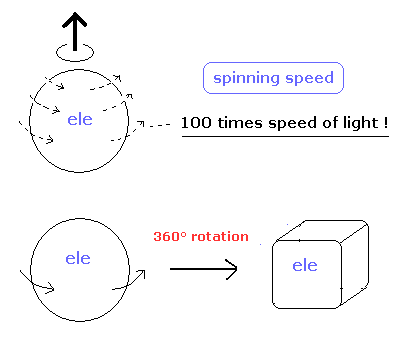If the electron spin means real rotating, its spinning speed must be more than 100 times speed of light, as shown on this page.
And the spinning electron can NOT return to its original configuration by one revolution.

( It needs two revolutions. This concept is impossible in this real world, as I said many times. )
So all physicists give up asking what the strange spin really is, which attitudes obstruct the development of science.

## Spin magnetic moment is equal to "Bohr magneton".

(Fig.10) Electron spin (= Bohr magneton ) = circular movement (= Bohr magneton ) ?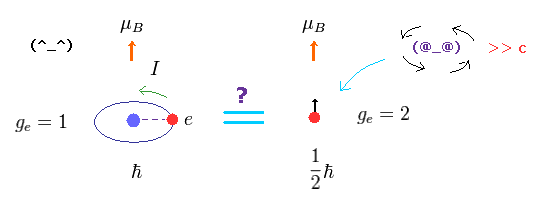As shown on this page, the magnetic moment means the current I × the area of the current loop (= πr2 ).
Surprizingly, the quantum mechanical spin also has the same magnetic moment (= Bohr magneton ) as Bohr's ground state circular orbit.

This trick exists in the electron spin g factor = "2".
As a result, the magnetic moment = g-factor × angular momentum = 2 × 1/2 = 1 (= Bohr magneton ).
The important point is that this Bohr magneton originates in Bohr's orbit, and can NOT be gotten from the quantum mechanics itself.

In conlusion, it is natural that all strange spins of various particles such as electron and nuclei are considered as some real rotations, because "Shut up and calculate !" can NEVER be a true theory.

## It is impossible to expect breakthrough giving up asking what.

(Fig.11) Two "Shut up and calculate !" obstruct the computer's development.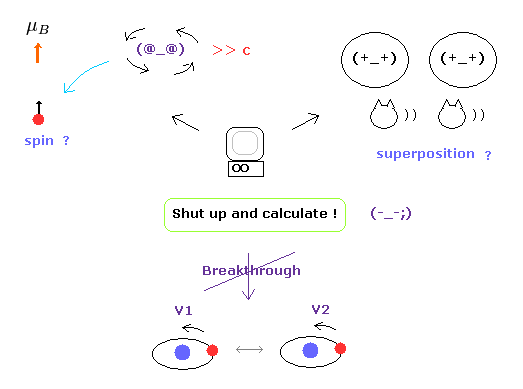As you may notice, it is completely inconsistent to aim at the development of quantum computers without asking deeper mechanisms such as wavefunctions and spins.

Unless we can manipulate each particle's position and velocity precisely, we cannot manipulate their various interactions and cannot achieve the development of the quantum computer itself.

Of course, knowing the position and velocity at the same time means uncertainty principle of quantum mechanics itself is wrong.

## Controlled-NOT gate using qubits = quantum computer.

### [ Trapped Be+ ion's energy states = qubits ? ]

(Fig.12) Hyperfine structure of trapped Be+ ion.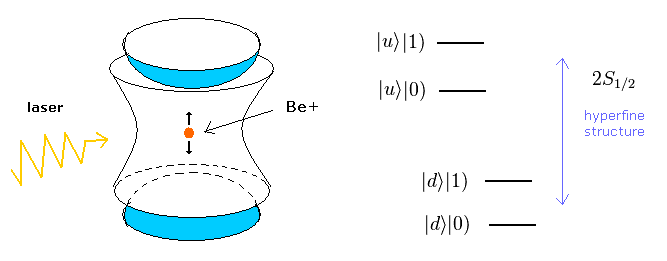First, we explain qubits based on Be+ ion which is oscillating trapped in Paul trap.
The quantum computers based on various ion trap have NOT been realized, so ONLY their theory developed a life of its own.

Considering nuclear spin ( or rotation ), 2s1/2 state of the valence electron of Be+ ion is divided into two hyperfine states ( up and down ). ( See this page. )
And we also produce small energy difference considering ion's motion and Coulomb interaction between ions (= "phonon" mode 1 or 0 ).

And they often insist two trapped Be+ ions can be entangled with each other.
But their distance is very short (= several µ m ).

So the entanglement of two ions is NOT true due to locality loophole.
( In short, they are just ocsillating classically by some irradiation, NOT related to mysterious entanglement. )

## Angular momentum zero ? = Shut up and calculate !

(Fig.13) Angular momentum zero (= s state ) → Shut up and calculate !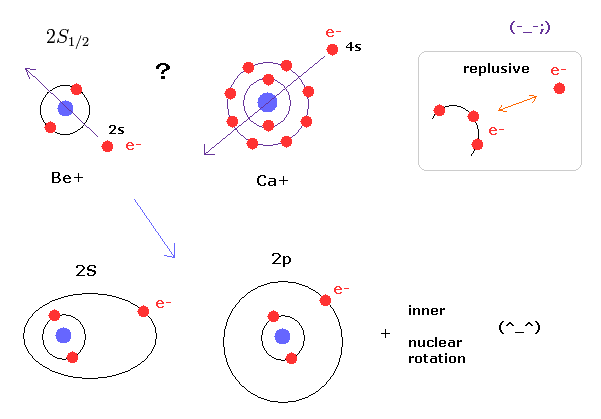The instant we suppose 2s1/2 state, which has NO orbital angular momentum, it means we accept "Shut up and calculate !" interpretation.
From a commonsense point of view, outer electron cannot penetrate inner electrons due to the strong repulsive Coulomb forces.

Instead of admitting this strage zero angular momentum, it is more natural that we think the outer electron is orbitting around the inner electrons. ( NOT penetrating them ! )
As shown on this page, if we consider elliptic and circular orbits, we can explain the energy difference between 2s and 2p states.

Furthermore, different from hydrogen atom, Be+ and Ca+ ions have inner electron's magnetic moment.
Natural orbits, inner electrons, and nuclear rotation can explain all spectrum results without doing "Shut up and calculate!".

(Eq.1) Four different states of trapped two Be+ ions.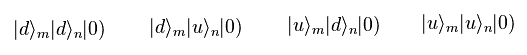First we prepare four different states, in which two Be+ ions of "m" and "n" are upper or down hyperfine energy levels.
And "phonon" energy mode of them is supposed to be zero.
( This phonon is very vague concept, you can consider it as some kinetic energies + Coulomb interactions between ions. )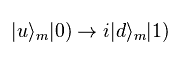Here we suppose if we irradiate "m" Be+ ion by right circularly polarized light (= π pulse ), its hyperfine state and phonon mode change like Eq.2.
Due to the π pulse, the phase factor "i" is added.
We cannot know the phase of Be+ ion itself. we only estimate it from the light and its irradiation time.
( You may consider this phase of ion as some state in oscillaltion. )

## Controlled-NOT gate. -- Be+ ion energy is too weak.

After irradiation of Eq.2, the four states of Eq.1 become
(Eq.3)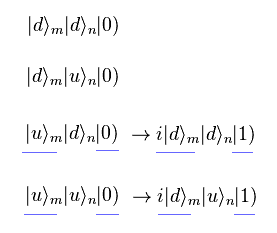And then we illuminate them by "2π" pulse laser, which irradiation time is twice π pulse.
So after the irradiation of "2π" pulse laser, the factor " -1 " is added to the phase of Be+ ion.
We suppose when we irradiate the "n" Be+ ion by left circularly polarized 2π pulse laser, the following change happens,
(Eq.4)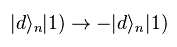Here we suppose phonon state is influencing both two Be+ ions.
As a result, after irradiation of Eq.4, the states of Eq.3 become
(Eq.5)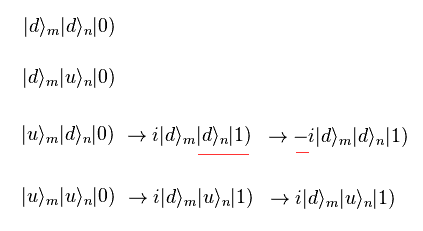And again we irradiate the "m" ion by right circularly polarized light ( π puse ).
So like Eq.2,
(Eq.6)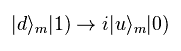As a result, the states of Eq.5 become
(Eq.7)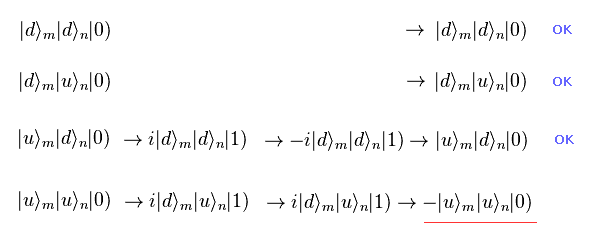As shown in Eq.7, only the phase of up and up hyperfine states becomes negative.
If we define the ± states of
(Eq.8)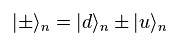From Eq.7 and Eq.8, we have
(Eq.9)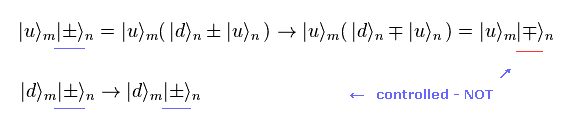As shown in Eq.9, only "up" state can change the sign of ±states.
This operation is called "controlled-NOT" gate, which is the basis of computers.

But of course, we cannot apply this manipulation of Be+ ions to usual computers due to various problems such as unstability and very short decoherence time ( about 0.1 second ).
So the present studies about the quantum computer have already reached their limits at this point.

## Superluminal entanglement is an illusion.

(Eq.10) Entanglement ?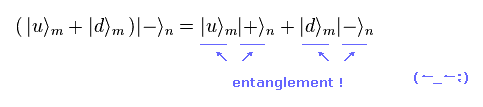Using Eq.9, we can generate the state of Eq.10.
In Eq.10, two pairs of ( up, + ) and ( down, - ) are generated.
So they insist these states are mysterious entanglement.
( In this case, up state is entangled with + state. )

But as I explained above, this state has NO relation with faster-than-light entanglement.
We only generate these states by usual classical manipulations.

It looks like they are trying to link everything to entanglement.
And the distance between Be+ ions is very short, locality loophole exists, in which ions can be controlled by usual classical irradiation.

## Other various quantum devices (= qubits ).

### [ Factorization of 15 = 5 × 3 is quantum computer ? ]

(Fig.14) Qubit molecule.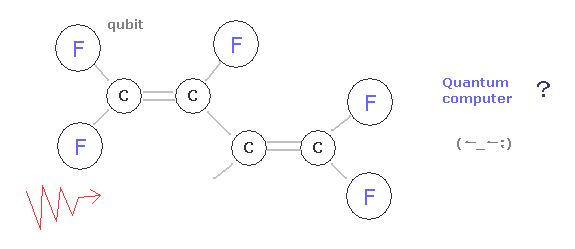The molecule of Fig.14 consists of several fluorines and carbons.
Due to the magnetic moment of each nucleus and interaction among electrons and nuclei, it is divided into various energy states, which intervals are very small.
Using these different energy states, nuclear magnetic resonance ( NMR ), and some algorism, they factorize 15 = 5 × 3.

But differernt from other quantum bits, they can get only average NMR spectrum informations from an enormous amount of molecules.
They cannot manipulate each quantum qubits (= atom ) independently, and don't use entanglement.

So this methods only use some different energy states and NMR, which has NO relation with superposition.
Due to various annoying motion of each atom, the factorization of 15 = 5 × 3 using 7 qubit molecule is their limit.

## Cavity QED has Nothing to do with entanglement.

(Fig.15) Cesium fine structure + polarized light.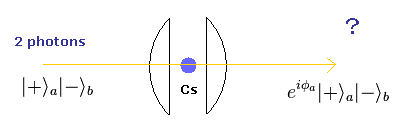In Fig.15, cesium (Cs) atom inside microcavity is irradiated by left (-) or right (+) polarized lights.
We set up the device in which only right polarized light tends to interact with cesium fine structre energy states.

So in this case, qubit is two energy levels of fine structure of Cs.
When we send two photons of following four patterns to cavity,
(Eq.11)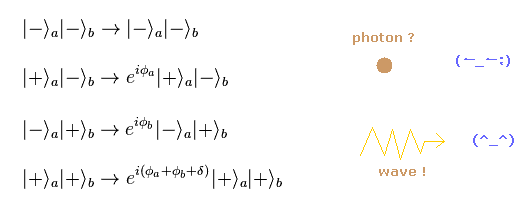We get various photon's phases depending on the interactions with Cs.
When δ becomes 180 degree, we can get controlled-NOT gate like upper Be+ ion.

But even this basic NOT gate cannot be realized in this method.
And the left or right circularly polarized lights are clearly classical electromagnetic waves, NOT photon particles.

## Any other qubits cannot realize quantum computer.

(Fig.16) Mach-Zehnder interferometer and Josephson junctions.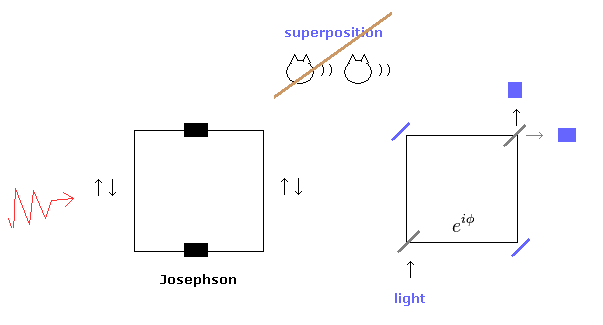In Fig.16 left, using some superconducting circuit including Josephson junctions, they try to get different energy levels.
These energy level difference can be gotten by manipulating directions and amounts of currents.
Though they often insist these states are "superposition", but they are just mixed states of different classical motions.

In Fig.16 right, they try to get various phases of electromagnetic waves using beam splitters in Mach-Zehnder interferometer.

They insist these states are quantum mechanical superpositions, but these are only interference patterns of classical electromagnetic waves.
Neither of these two methods has been realized as quantum computer at all.

## Quantum computers based on illusion would NEVER be realized.

(Fig.17) Parallel-worlds = superposition is just an illusion !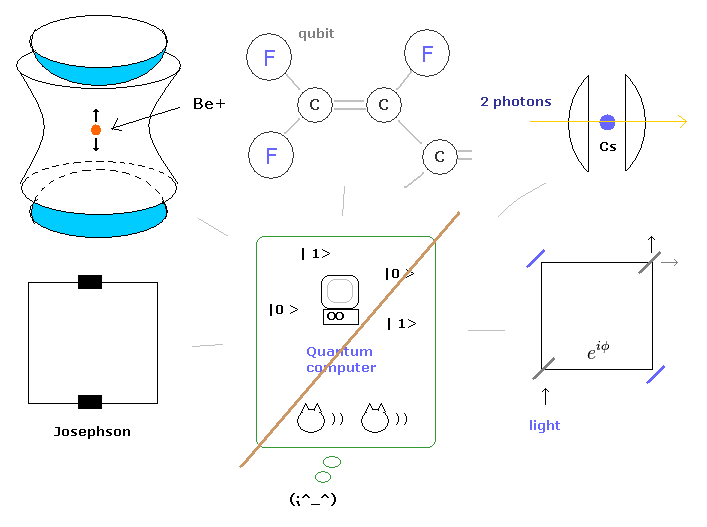As I showed in the above sections, NO quantum qubits utilize the powers specific to quantum "superposition".
So it seems that only fascinating words such as "parallel computing" and "entanglement" have attracted people's attentions without reality.

Studies about the present quantum computers based on "superposition" have reached an dead end.
This serious fact indicates that they are aiming at essentially wrong concept and just a illusion.

## Cooper pair really exists ?

(Fig.18) "Cooper pair" really exists ??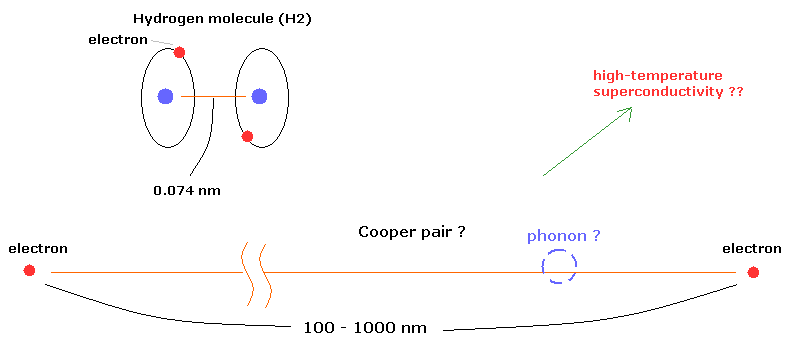According to the present condensed matter physics, it is said that a Cooper pair is two electrons that are bound together at low temperature through very vague existence of "phonons".
Surprisingly, the distance between the two electrons in the Cooper pairs is about 100- 1000 nanometer (nm) !

For example, in the hydrogen molecule (H2), the distance between two nuclei is about 0.073 nm.
How can these two electrons bind stably to each other in the superconductor ? This is strange.

According to the standard interpretation, positive ions (nuclei) are said to be related to this very long binding as a quasiparticles such as phonons.
( But I think, this very long binding is very unnatural. How do you think about it ? )

According to BCS theory, under some low temperature, the Cooper pair is formed in the superconductivity.
So the present BCS theory can not explain high-temperature superconductivity.

## Bohr model and de Broglie's wave can explain magnetic flux quantum.

(Fig.19)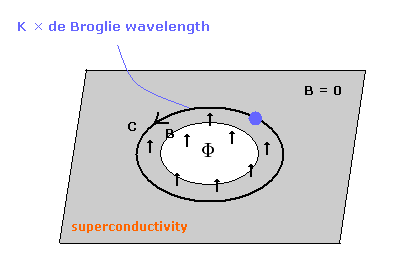One of the most important facts which support the Cooper pair is magnetic flux quantum (= Φ0 ).
It is known that the magnetic flux (Φ) is quantized, when it passes through a superconductor ring.

(Eq.12) Quantized magnetic flux.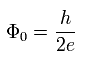As shown on this page, they try to explain this quantized magnetic flux using Cooper pair.
Of course, strange spin is also one of the reasons why they need Cooper pair.

Because if spin 1/2 fermions exist as they are, they cannot show boson-like nature.
So Cooper pair can cancel this spin 1/2 effect out, they insist.
But as I show in Fig.18, this Cooper pair is unrealistically too long.

## One orbit = an integer times de Broglie wavelength.

Here we suppose the electron is rotating around near the hole of Fig.19 to cancel the magnetic field.
When the centrifugal force is equal to the Lorentz force,
(Eq.13)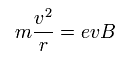When one round of the orbit is equal to an integer (= K ) times de Broglie's wavelength,
(Eq.14)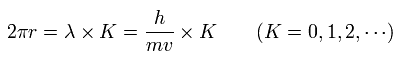where λ = h/mv.

From Eq.13 and Eq.14, we have
(Eq.15)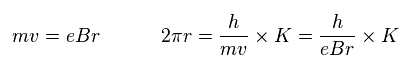So,
(Eq.16)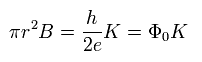Eq.16 means the magnetic flux (Φ) passing through the hole of Fig.19
( πr2 is the area of the hole. )
As a result, we can get the magnetic flux quantum of superconductor using "real" de Broglie waves.

(Eq.12) Quantized magnetic flux.And Cooper pair is not needed here.
Of course, if Cooper pair does not exist, the problem of high-temperature superconductivity never happen from the beginning.
( They try to solve this problem using conformal field theory of the string. But these methods have NO reality. )

## Josephson effect by Bohr model.

The Josephson effect is the phenomenon of electric current across two weakly coupled superconductors, separated by a very thin insulating barrier.
In this arrangement, the two superconductors are linked by a non-conducting barrier, which is called "Josephson junction".
In 1962, Brian David Josephson prediced this effect, and it is important in the quantum-mechanical circuits, such as SQUIDs.

The Josephson junction barrier is very thin, so if we apply the weak stimulus, the electric current can pass through it.
But even in the no magnetic field such as superconductor, the electric current occur in this barrier. (Quantum tunneling.)
If we can explain this quantum tunnel effect by de Broglie's waves, this means that Bohr model can explain Josephson effect and Quantum tunneling.

(Fig.20) Josephson juction.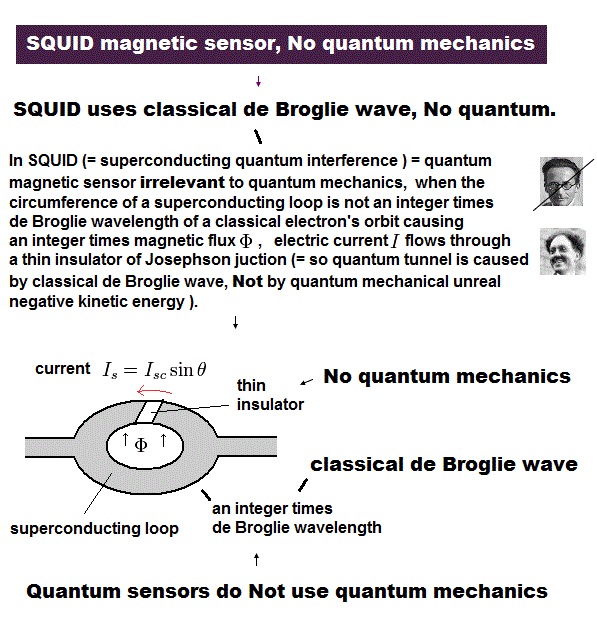The DC Josephson effect is the phenomenon of a direct current crossing from the insulator in the absence of any external electromagnetic field, owing to tunneling.
(Of course, to make the magnetic field zero in the superconductor, the electric current only near the hole exists to cancel Φ.)

This DC Josephson current is proportional to the sine of the phase difference across the insulator, as follows,
(Eq.17)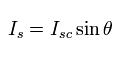where the phase difference θ can be expressed as
(Eq.18)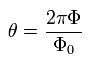Eq.17 and Eq.18 mean that when the magnetic flux (Φ) in the hole is an integer times the magnetic flux quantum, the phase difference becomes zero, and the Josephson currenct doesn't occur.
So we can insist that the Josephson juction is influenced by de Broglie's waves of electrons near the hole.
The quantum tunnel effect is caused by de Broglie's wave itself.

With a fixed voltage V across the junction, the phase will vary linearly with time, and the current becomes an AC current.
(AC Josephson effect.)
According to Maxwell equation, the voltage of the ring in Fig.20 can be expresses as
(Eq.19)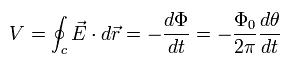where Eq.18 is used.

Integrating Eq.19 by time t, we can get
(Eq.20)Substituting Eq.20 into Eq.17, we can get AC Josephson current of
(Eq.21)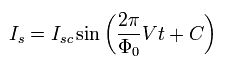So the frequency of Eq.21 is
(Eq.22)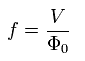The frequency of Eq.22 can be confirmed by the microwave, which synchronizes with the AC Josephson current. (Shapiro step.)
This measured voltage (V) is used to give the definition of a volt.
And the Josephson effect can give the correct Planck constant (h), which is included in Φ0.
(Of course, this Planck constant originates from de Broglie's relation of the electron.)

## Quantum Zeno effect is real ?

### [ Quantum Zeno ( paradox ) effect can be explained by classical mechanics. ]

(Fig.21) A flying arrow "stops" by repeated measurements ??Quanum Zeno effect suggested that a flying arrow stops, when we try to measure its position at very short time intervals.
Though this arrow is actually flying and moving (= paradox ).

It is said that this mysterious quantum effects were actually observed in some experiments.
But this section proves that these Zeno effects can be explained by usual classical mechanics, and they do NOT show quantum mechanical validity at all.

(Eq.23)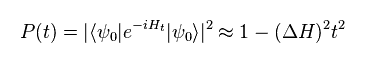In Eq.23, the initial state changes after short time (= t ) by time evolution.
The probability of this transition is P(t).

(Eq.24)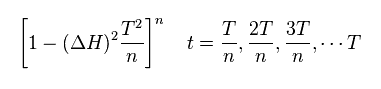We measure this state n times during T.
So the measuring time interval is T/n.
Eq.24 shows the probability after n times measurements.

(Eq.25)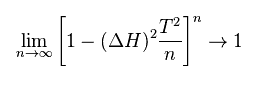If we make this interval infinitesimal by measureing it inifnite times.
As shown in Eq.25, as n is close to infinity, the probability of the transition is close to 1.
It means the transition (= change ) does NOT happen by infinite measurements at very short time intervals !

But is it really happening in this real world ??

(Fig.22) Experimental results of Zeno effects ?   Really ??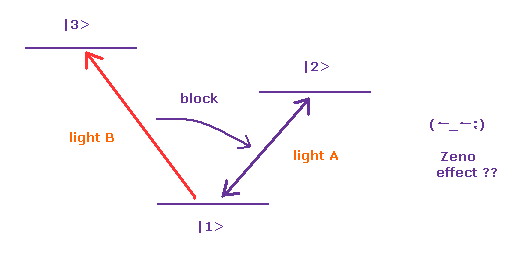It is said that the quantum Zeno effect was first observed experimentally in 1989 in Be+ ions trapped by electromagnetic fields.
By irradiating some light (= light A) to Be ion, its hyperfine states change between two energy levels (= 1 and 2 ).

But if we illuminate this ion by another light B ( 1 → 3 ), just after the irradiation of light A, the transition from 1 to 2 is inhibited.
So they insist this is quantum mechanical Zeno effect.

But as you feel, in this experiement, they just illuminate the ion by the light B before the transition from 1 to 2 is completed. (= So these are just classical phenomena ! )
Because the time interval between irradiations of light A and B is too short.

(Fig.23) Nitrogen-vacancy (NV) centre = quantum computer ??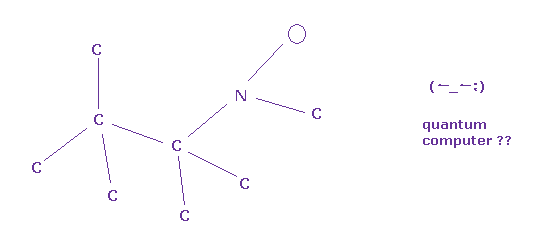In nitrogen-vacency (NV) centre, one carbon is replaced by the nitrogen, and a atom is vacent next to this nitrogen inside diamond.
This NV centre is stable and includes various energy states related to "spin" at room temperature.
So they try to use this NV centre as quantum computer ( bit ).

But as I explained in this page, quantum computers and entanglements are just imaginary things.
Actually, the quantum computer and entanglment are NEVER useful for our daily lives, though they have been doing these experiments extremely many times.
In fact, physicists ( of quantum computer ) themselves don't think quantum computers can be realized in the future.

### [ Spin does NOT exist. they are just "energy" states. ]

(Fig.24) Point particle electron can be spin-polarized ??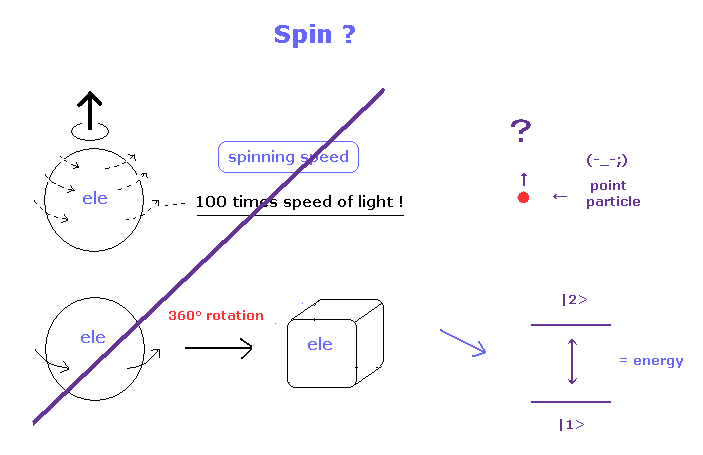As shown on this page, it is impossible that a very tiny electron can spin at the angular momentum of 1/2 ħ.
Because the electron must rotate much faster than the light speed c.
Instead of angular momentum 0 state (= s ), Bohr-Sommerfeld model has additional circular motion of electron.
Considering electron magnetic moment is equal to Bohr magneton, spin means just classical circular orbit of Bohr model.

And it is impossible that point like electron can clearly turn in some direction.
How can the point particle with no size be spin-polarized without rotation ? ( Impossible ! )

They often use this word of "spin" in various papers.
But of course, in the actual experiments, they cannot see (rotating) spin itself, they just measure the estimated energy states.
As shown on this page, spin-orbit energy difference is just equal to Bohr-Sommerfeld fine structure without spin.
This is very strange.

(Fig.25) Nitorogen-vacancy (NV) centre = Zeno effect ? Really ??Also in the case of NV centre, they use the same mechanism of ions in Fig.22.
Green laser (= measurement ) only blocks the transition between G0 and G1 by microwave (= MW ).
Because their time intervals are very short (= ns ).

In conclusion, quantum Zeno (paradox) effect does NOT show quantum mechanical phenomena.
In these experimets, one laser just inhibits another laser's effect. That's all.
So they can be explained by usual classical mechanics.
And they should not use this Zeno effect as the victory of wrong quantum mechanics, I think.2013/1/29 updated. Feel free to link to this site.